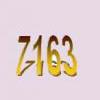#### You may also like### Some Cubes

The sum of the cubes of two numbers is 7163. What are these numbers?### Em'power'ed

Find the smallest numbers a, b, and c such that: a^2 = 2b^3 = 3c^5 What can you say about other solutions to this problem?### Sixational

The nth term of a sequence is given by the formula n^3 + 11n . Find the first four terms of the sequence given by this formula and the first term of the sequence which is bigger than one million. Prove that all terms of the sequence are divisible by 6.

# Old Nuts

##### Age 16 to 18 Challenge Level:

This can be solved by just working systematically with the information, thinking about which numbers are divisible by 4 and 3, and testing the possibilities.

Modulus arithmetic also provides an efficient way to solve the problem.